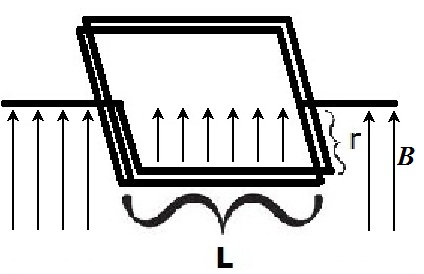Calculation of Back-EMF

In class, we calculate an estimate the back-EMF produced by a motor with a 6-turn square coil, when it is spinning at its maximum frequency, and when one arm of the coil is at its closest position to the magnet.

We make the following simplifying assumptions:

• The magnetic field B has constant strength and direction over the arm closest to the magnet.
• The magnetic field is 0 over the farther arm.

This gives us the following picture:Derivation

To derive a formula for the back EMF, we start with the equation for the magnetic force on a charge:

FB = qvBsinθ

Dividing both sides by the charge, q, gives the magnetic force per unit charge:

FB/q = vBsinθ

If the force acts over a distance D, then the work per unit charge is:

D*FB/q = DvBsinθ

This has units of Joules per Coulomb (J/C). That is, it has units of Voltage. This formula gives us the voltage induced by motion with velocity v through a magnetic field B. With this formula, we can calculate the voltage induced accross the lower arm of the coil as it rotates through the magnetic field. This is the back-EMF:

Vback = DvBsinθ

The angle θ between v and B at this point in time is π/2, by the assumption of constant B, so sinθ = 1. v = 2π*f*r, where f is the frequency of the motor's rotation in Hz. D = n*L, where L is the length of the arm of the coil and n is the number of turns. Making these substitutions, we obtain:

Values

The magnetic field B was measured before the demonstration at the point where the coil is closest to the magnet. If the values of L and r are given in meters, then B should be given in Teslas. L and r were measured with a ruler. n, the number of coil turns, was recorded when the coil was wound. f was measured in class using a stroboscope.

By multiplying all these values together, we obtain our estimate for the peak back-EMF.

Further Refinements

This is only the peak back-EMF. A time-averaged back-EMF for the entire cycle could be obtained by integrating over θ. In addition, the assumption of constant B will not hold well at other points in the rotation; the field strength drops off rapidly as the arm of the coil moves away from the magnet, so this must be taken into account.